# C++语言的表达式模板：表达式模板的入门性介绍

### 阶乘——编译时计算的第一个例子

int factorial(int n)
{
return (n == 0 ? 1 : n * factorial(n - 1));
}


cout << factorial(4) << endl;

template <int n>
struct factorial
{
enum { ret = factorial<n-1>::ret * n };
};



template <typename T> class X {...};

cout << factorial<4>::ret <<endl;

template <>
struct factorial<0>
{
enum { ret = 1 };
};


### 开平方根——编译时计算的又一个例子

int array[ceil(sqrt(N))];

int array[Root<10>::ret];


Root的代码如下：

template <size_t N, size_t Low = 1, size_t Upp = N> struct Root
{
static const size_t ret = Root<N, (down ? Low : mean + 1),
(down ? mean : Upp)>::ret;

static const size_t mean = (Low + Upp) / 2;
static const bool down = ((mean * mean) >= N);
};


• 需要开方的数
• 预期平方根的上界和下界。默认值是1和N。平方根必然是介于1和N之间的某个数。

template <size_t N, size_t Mid>
struct Root<N, Mid, Mid>
{
static const size_t ret = Mid;
};


Root<10, 1, 10>;
Root<10, 1, 5>;
Root<10, 4, 5>;
Root<10, 4, 4>;

### 表达式模板

int a = {1, 100, 0, -1};
int b = {2, 2, 2, 2};
cout << dot<4>(a, b) <<endl;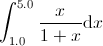template <typename ExprT>
double integrate(ExprT e, double from, double to, size_t n)
{
double sum = 0;
double step = (to - from) / n;

for(double i = from + step / 2; i < to; i += step)
sum += e.eval(i);

return step * sum;
}


### 点乘（I）——表达式模板的第一个应用

• 叶结点定义组合体中个体对象的行为。
• 组合体定义叶结点集合的行为。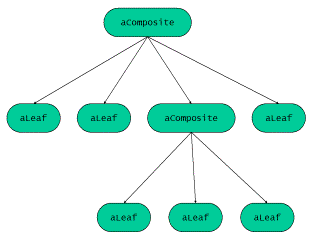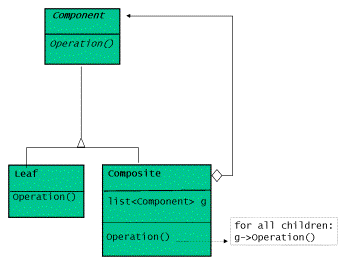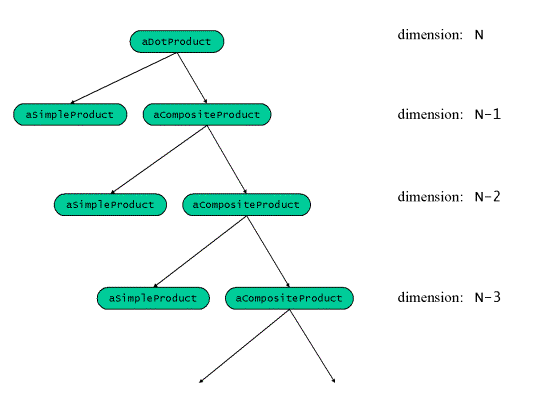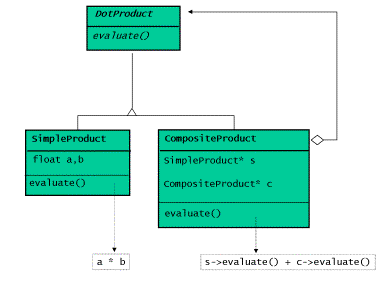template <typename T> class DotProduct
{
public:
virtual ~DotProduct () {}
virtual T eval() = 0;
};


template <typename T>
class CompositeDotProduct  : public DotProduct<T>
{
public:
CompositeDotProduct (T* a, T* b, size_t dim) :
s(new SimpleDotProduct<T>(a, b)),
c((dim == 1) ? 0 : new CompositeDotProduct<T>(a + 1, b + 1, dim - 1))
{}

virtual ~CompositeDotProduct ()
{
delete c;
delete s;
}

virtual T eval()
{
return ( s->eval() + ((c) ? c->eval() : 0));
}

protected:
SimpleDotProduct<T>* s;
CompositeDotProduct<T>* c;
};


template <typename T>
class SimpleDotProduct  : public DotProduct<T>
{
public:
SimpleDotProduct (T* a, T* b) : v1(a), v2(b)
{}

virtual T eval()
{
return (*v1)*(*v2);
}
private:
T* v1;
T* v2;
};


template <typename T>
T dot(T* a, T* b, size_t dim)
{
return (dim == 1) ?
SimpleDotProduct<T>(a, b).eval() :
CompositeDotProduct<T>(a, b, dim).eval();
}


int a = {1, 100, 0, -1};
int b = {2, 2, 2, 2};
cout << dot(a, b, 4);

SimpleDotProduct<T>::SimpleDotProduct (T* a, T* b) : v1(a), v2(b)
{}

virtual T SimpleDotProduct<T>::eval()
{
return (*v1)*(*v2);
}


T SimpleDotProduct::eval(T* a, T* b, size_t dim)
{
return (*a)*(*b);
}


template <typename T>
class CompositeDotProduct : public DotProduct <T>
{
public:
virtual T eval(T* a, T* b, size_t dim)
{
return  SimpleDotProduct<T>().eval(a,b,dim) + ((dim==1) ?
0 : CompositeDotProduct<T>().eval(a+1,b+1,dim-1));
} };

template <typename T>
class SimpleDotProduct : public DotProduct <T>
{
public:
virtual T eval(T* a, T* b, size_t dim)
{
return (*a)*(*b);
}
};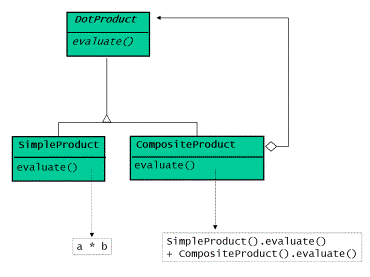### 点乘（II）——编译时计算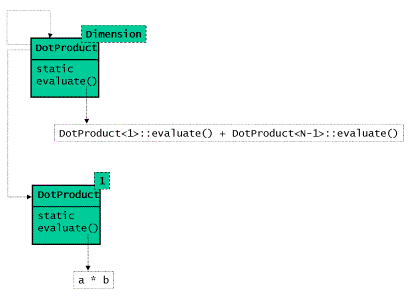template <size_t N, typename T>
class DotProduct
{
public:
static T eval(T* a, T* b)
{
return  DotProduct<1,T>::eval(a,b) + DotProduct<N-1,T>::eval(a+1,b+1);
}
};

template <typename T>
class DotProduct<1,T>
{
public:
static T eval(T* a, T* b)
{
return (*a)*(*b);
}
};


template <size_t N, typename T>
inline T dot(T* a, T* b)
{
return DotProduct<N,T>::eval(a, b);
}

int a = {1, 100, 0, -1};
int b = {2, 2, 2, 2};
cout << dot<4>(a,b);


• dot(a, b, 4)等价于CompositeDotProduct<int>().eval(a, b, 4)，递归式的引发如下函数在运行时的调用：
SimpleDotProduct<int>().eval(a, b, 1);
CompositeDotProduct<int>().eval(a + 1, b + 1, 3);
SimpleDotProduct<int>().eval(a + 1, b + 1, 1);
CompositeDotProduct<int>().eval(a + 2, b + 2, 2);
SimpleDotProduct<int>().eval(a + 2, b + 2, 1);
CompositeDotProduct<int>().eval(a + 3, b + 3, 1);
SimpleDotProduct<int>().eval(a + 3, b + 3, 1);


• dot<4>(a, b)通过计算DotProduct<4, int>::eval(a, b)，从而递归式的引发下列模板依次实例化展开：
DotProduct<4, size_t>::eval(a, b);
DotProduct<1, size_t>::eval(a, b) + DotProduct<3, size_t>::eval(a + 1, b + 1);
(*a) * (*b) + DotProduct<1, size_t>::eval(a + 1, b + 1) + DotProduct<2, size_t>::eval(a + 2, b + 2);
(*a) * (*b) + (*a + 1) * (*b + 1) + DotProduct<1, size_t>::eval(a + 2, b + 2) + DotProduct<1, size_t>::eval(a + 3, b + 3);
(*a) * (*b) + (*a + 1) * (*b + 1) + (*a + 2) * (*b + 2) + (*a + 3) * (*b + 3)

### 算术表达式——表达式模板的另一个应用

• 叶结点是一个终点表达式（terminal expression）。
• 组合体是一个非终点表达式（nonterminal expression）。
• 通过解释表达式树和其中包含的表达式来进行求值。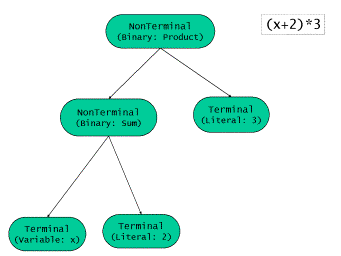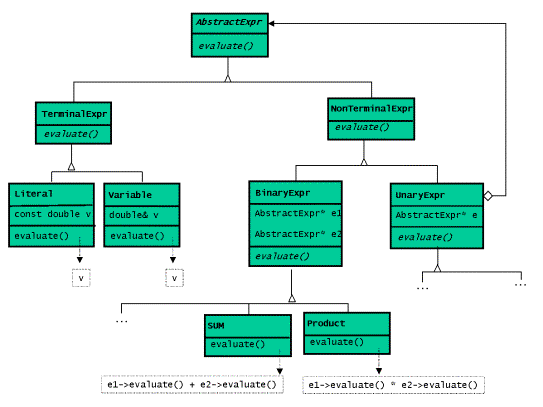class AbstractExpr
{
public:
virtual double eval() const = 0; };

class TerminalExpr : public AbstractExpr
{ };

class NonTerminalExpr : public AbstractExpr
{ };

class Literal : public TerminalExpr
{
public:
Literal(double v) : _val(v)
{}

double eval() const
{
return _val;
}

private:
const double _val;
};

class Variable : public TerminalExpr
{
public:
Variable(double& v) : _val(v)
{}

double eval() const
{
return _val;
}

private:
double& _val;
};

class BinaryExpr : public NonTerminalExpr
{
protected:
BinaryExpr(const AbstractExpr* e1, const AbstractExpr* e2) : _expr1(e1),_expr2(e2)
{}

virtual ~BinaryExpr ()
{
delete const_cast<AbstractExpr*>(_expr1);
delete const_cast<AbstractExpr*>(_expr2);
}

const AbstractExpr* _expr1;
const AbstractExpr* _expr2;
};

class Sum : public BinaryExpr
{
public:
Sum(const AbstractExpr* e1, const AbstractExpr* e2) : BinExpr(e1,e2)
{}

double eval() const
{
return _expr1->eval() + _expr2->eval();
}
};

//...


void someFunction(double x)
{
Product expr(new Sum(new Variable(x), new Literal(2)), new Literal(3));
cout << expr.eval() <<endl;
}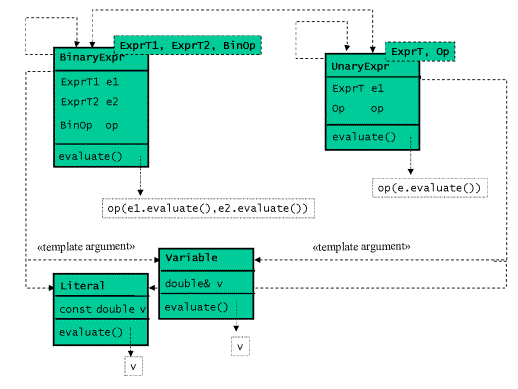class Literal
{
public:
Literal(const double v) : _val(v)
{}

double eval() const
{
return _val;
}

private:
const double _val;
};

class Variable
{
public:
Variable(double& v) : _val(v)
{}

double eval() const
{
return _val;
}

private:
double& _val;
};

template <typename ExprT1, typename ExprT2, typename BinOp>
class BinaryExpr
{
public:
BinaryExpr(ExprT1 e1, ExprT2 e2,BinOp op=BinOp()) :
_expr1(e1),_expr2(e2),_op(op)
{}

double eval() const
{
return _op(_expr1.eval(),_expr2.eval());
}

private:
ExprT1 _expr1;
ExprT2 _expr2;
BinOp  _op;
};

//...


UnaryExpr的类模板与BinaryExpr的类模板相似。对于实际操作，我们可以使用已经编写好 的STL的仿函数类plus，minus，等等，或者我们也可以自行编写。一个用来表示和的二元 表达式的类型是BinaryExpr<ExprT1, ExprT2, std::plus<double>>。（译注9）这样的类 型使用起来较为复杂，因此我们写一个产生函数，方便以后的使用。

#### 产生函数

template <typename T1, typename T2>
class pair
{
public:
pair(T1 t1, T2 t2);
// ...
};

template <typename T1, typename T2>
pair<T1,T2> make_pair(t1 t1, T2 t2)
{
return pair<T1,T2>(t1, t2);
}


pair< pair<string,complex<double>>, pair<string,complex<double>>>(
pair<string,complex<double> >("origin", complex<double>(0,0)),


 make_pair(make_pair("origin", complex<double>(0,0)), make_pair("saddle", aCalculation()))


template  <typename ExprT1, typename ExprT2>
BinaryExpr<ExprT1,ExprT2,plus<double>> makeSum(ExprT1 e1, ExprT2 e2)
{
return BinaryExpr<ExprT1,ExprT2,plus<double> >(e1,e2);
}

template  <typename ExprT1, typename ExprT2>
BinaryExpr <ExprT1,ExprT2,multiplies<double>> makeProd(ExprT1 e1, ExprT2 e2)
{
return BinaryExpr<ExprT1,ExprT2,multiplies<double> >(e1,e2);
}


void someFunction (double x)
{
BinaryExpr< BinaryExpr < Variable, Literal, plus<double> >, multiplies<double>>
expr = makeProd (makeSum (Variable(x), Literal(2)), Literal(3));
cout << expr.eval() << endl;
}


cout << makeProd(makeSum(Variable(x),Literal(2)),Literal(3)).eval() << endl;


Product expr(new Sum(new Variable(x),new Literal(2)), new Literal(3)).eval()

### 表达式模板的进一步应用

makeProd(makeSum(Variable(x), Literal(2)), Literal(3)).eval() 

makeProd(makeSum(Variable(x), Literal(2)), Literal(3))

((Variable(x) + Literal(2)) * Literal(3))

template <typename ExprT1, typename ExprT2>
BinaryExpr<ExprT1, ExprT2, plus<double>> operator+(ExprT1 e1, ExprT2 e2)
{
return BinaryExpr<ExprT1, ExprT2, plus<double>>(e1,e2);
}


plus<double>>(x, 2)的匿名对象。然而我们所期望的类型并非如此：需要的是BinaryExpr <Variable, Literal, plus<double>>类型的对象。可是，自动模板参数类型推导并不知道x是一个变量，而2是一个常量。编译器只能检查传递给函数的参数类型，从而从x中推导出double类型，从2中推导出int类型。

void someFunction (double x)
{
Variable v = x;
cout << ((v + 2) * 3).eval() << endl;
}

#### Traits *TRAITS*

C++ STL中有多个traits的例子，字符traits就是其中之一。读者大约知道标准库中的string类其实是一个类模板，这个类模板的参数是具体的字符类型。这就使得string可以处理普通的字符和宽字符。实际上，string类的实际名称是basic_string。basic_string可以通过实例化来接受任何类型的字符，而不仅仅是上述提及的两种。倘若有人需要保存以Jchar定义的日文字符，那么他就可以用basic_string模板实现：basic_string<Jchar>。

template <typename ExprT>
struct exprTraits
{
typedef ExprT expr_type;
};

template <>
struct exprTraits<double>
{
typedef Literal expr_type;
};

template <>
struct exprTraits<int>
{
typedef Literal expr_type;
};

//...


template <typename ExprT1, typename ExprT2, typename BinOp>
class BinaryExpr
{
public:
BinaryExpr(ExprT1 e1, ExprT2 e2,BinOp op=BinOp()) :
_expr1(e1), _expr2(e2), _op(op)
{}

double eval() const
{
return _op(_expr1.eval(),_expr2.eval());
}

private:
exprTraits<ExprT1>::expr_type _expr1;
exprTraits<ExprT2>::expr_type _expr2;
BinOp  _op;
};


template <class ExprT> double eval(ExprT e)
{
return e.eval();
}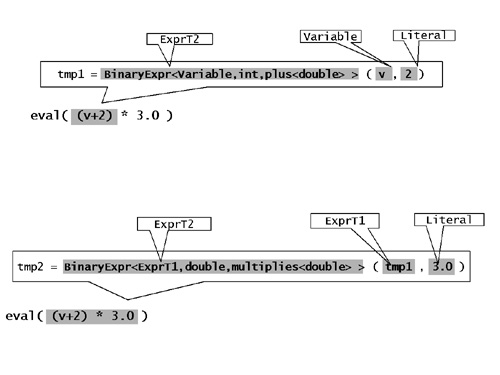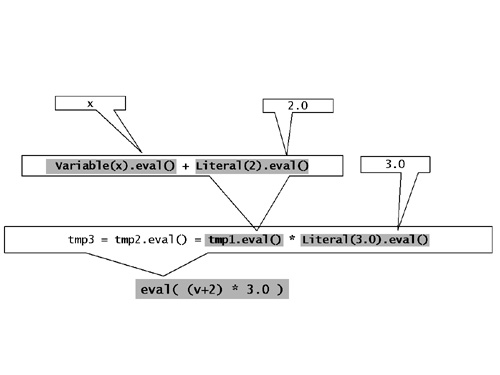### 表达式对象的反复计算

template <class ExprT>
double integrate (ExprT e,double from,double to,size_t n)
{
double sum = 0;
double step = (to - from) / n;
for (double i = from + step / 2; i < to; i += step)
sum += e.eval(i);
return step * sum;
}Identity<double> x;
cout << integrate(x / (1.0 + x), 1.0, 5.0, 10) << endl;

class Literal
{
public:
Literal(double v) : _val(v)
{}

double eval(double) const
{
return _val;
}

private:
const double _val;
};

template<class T> class Identity
{
public:
T eval(T d) const
{
return d;
}
};

template <class ExprT1,class ExprT2, class BinOp> class BinExpr
{
public:
double eval(double d) const
{
return _op(_expr1.eval(d),_expr2.eval(d));
}
};

//...


double sigma = 2.0;
double mean = 5.0;
const double Pi = 3.141593;
cout << integrate(
1.0 / (sqrt(2 * Pi) * sigma) * exp(sqr(x - mean) / (-2 * sigma * sigma)),
2.0, 10.0, 100) << endl;


template  <typename ExprT>
UnaryExpr<ExprT, double(*)(double)> sqrt(const ExprT& e)
{
return UnaryExpr<ExprT, double(*)(double)>(e, ::std::sqrt);
}

template  <typename ExprT>
UnaryExpr<ExprT, double(*)(double)> exp(const ExprT& e)
{
return UnaryExpr<ExprT,double(*)(double)>(e,::std::exp);
}

// ...


list<int> l;
Identity<int> x;
count_if(l.begin(), l.end(), x >= 10 && x <= 100);

### 参考文献

04-18
01-03314
04-21227
08-177609
04-19162
03-2049
04-22545
11-24699
11-21106
10-0756
11-27693
04-15403
10-24356
09-17125
10-1491

### “相关推荐”对你有帮助么？

•非常没帮助
•没帮助
•一般
•有帮助
•非常有帮助被折叠的  条评论 为什么被折叠?到【灌水乐园】发言点击重新获取扫码支付1.余额是钱包充值的虚拟货币，按照1:1的比例进行支付金额的抵扣。
2.余额无法直接购买下载，可以购买VIP、C币套餐、付费专栏及课程。余额充值